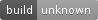## simple_parallel

Straight-forward functions and types for basic data parallel operations, including parallel maps, for loops and thread pools

### 8 unstable releases(3 breaking)

Uses old Rust 2015

 0.3.0 Oct 11, 2015 Aug 3, 2015 May 24, 2015 Apr 20, 2015 Feb 19, 2015

#58 in #parallel

Used in 3 crates

MIT/Apache

36KB
522 lines

# `simple_parallel`Straight-forward functions and types for basic data parallel operations.

### `lib.rs`:

Straight-forward functions and types for basic data parallel operations.

This library provides a few building blocks for operating on data in parallel, particularly iterators. At the moment, it is not designed to be robust or eke out every last drop of performance, but rather explore some ways in which Rust's type system allows for some fairly fancy things to be written with a guarantee of safety, all without a garbage collector.

The core design is to simply allow for operations that could occur on a single thread to execute on many, it is not intending to serve as a hard boundary between threads; in particular, if something (a `panic!`) would take down the main thread when run sequentially, it will also take down the main thread (eventually) when run using the functions in this library.

On the point of performance and robustness, the top level functions do no thread pooling and so everything essentially spawns a new thread for each element, which is definitely suboptimal for many reasons. Fortunately, not all is lost, the functionality is designed to be as generic as possible, so the iterator functions work with many many iterators, e.g. instead of executing a thread on every element of a vector individually, a user can divide that vector into disjoint sections and spread those across much fewer threads (e.g. the `chunks` method).

Further, the thread pooling that does exist has a lot of synchronisation overhead, and so is actually rarely a performance improvement (although it is a robustness improvement over the top-level functions, since it limits the number of threads that will be spawned).

Either way, this is not recommended for general use.

# Usage

``````[dependencies]
simple_parallel = "0.3"
``````

The latest development version can be obtained on GitHub.

# Examples

Initialise an array, in parallel.

``````# fn foo() {
let mut data = [0; 10];
// fill the array, with one thread for each element:
simple_parallel::for_(data.iter_mut().enumerate(), |(i, elem)| {
*elem = i as i32;
});
# }

# let mut data = [0; 10];
let mut pool = simple_parallel::Pool::new(4);
pool.for_(data.iter_mut(), |elem| *elem *= 2);
``````

Transform each element of an ordered map in a fancy way, in parallel, with `map` (`map` ensures the output order matches the input order, unlike `unordered_map`),

``````extern crate crossbeam;
extern crate simple_parallel;

use std::collections::BTreeMap;

let mut map = BTreeMap::new();
map.insert('a', 1);
map.insert('x', 55);

crossbeam::scope(|scope| {
// (`IntoIterator` is used, so "direct" iteration like this is fine.)
let par_iter = simple_parallel::map(scope, &map, |(&c, &elem)| {
let mut x = elem  * c as i32;
// ... something complicated and expensive ...
return x as f64
});

// the computation is executing on several threads in the
// background, so that elements are hopefully ready as soon as
// possible.

for value in par_iter {
println!("I computed {}", value);
}
});
``````

Sum an arbitrarily long slice, in parallel, by summing subsections and adding everything to a shared mutex, stored on the stack of the main thread. (A parallel fold is currently missing, hence the mutex.)

``````use std::sync::Mutex;

// limit the spew of thread spawning to something sensible
const NUM_CHUNKS: usize = 8;

fn sum(x: &[f64]) -> f64 {
// (round up)
let elements_per_chunk = (x.len() + NUM_CHUNKS - 1) / NUM_CHUNKS;

let total = Mutex::new(0.0);
simple_parallel::for_(x.chunks(elements_per_chunk), |chunk| {
// sum up this little subsection
let subsum = chunk.iter().fold(0.0, |a, b| a + *b);
*total.lock().unwrap() += subsum;
});

}
``````

Alternatively, one could use a thread pool, and assign an absolute number of elements to each subsection and let the pool manage distributing the work among threads, instead of being forced to computing the length of the subsections to limit the number of threads spawned.

``````use std::sync::Mutex;

// limit the spew of thread spawning to something sensible
const ELEMS_PER_JOB: usize = 1_000;

fn pooled_sum(pool: &mut simple_parallel::Pool, x: &[f64]) -> f64 {
let total = Mutex::new(0.0);
pool.for_(x.chunks(ELEMS_PER_JOB), |chunk| {
// sum up this little subsection
let subsum = chunk.iter().fold(0.0, |a, b| a + *b);
*total.lock().unwrap() += subsum;
});

}
``````

A sketch of a very simple recursive parallel merge-sort, using `both` to handle the recursion. (A working implementation may really need some temporary buffers to mangle the data, but the key point is `both` naturally running things in parallel.)

``````/// Merges the two sorted runs `left` and `right`.
/// That is, after `merge(left, right)`,
///
///    left <= left <= ... <= left[last] <= right <= ...
fn merge<T: Ord>(left: &mut [T], right: &mut [T]) {
// magic (but non-parallel, so boring)
}

fn parallel_merge_sort<T: Ord + Send>(x: &mut [T]) {
// base case
if x.len() <= 1 { return }

// get two disjoint halves of the `x`,
let half = x.len() / 2;
let (left, right) = x.split_at_mut(half);
// and sort them recursively, in parallel
simple_parallel::both(&mut *left, &mut *right, |v| parallel_merge_sort(v));

// now combine the two sorted halves
merge(left, right)
}
``````

The `examples` folder contains more intricate example(s), such as a parallel fast Fourier transform implementation (it really works, and the parallelism does buy something... when tuned).

~14KB